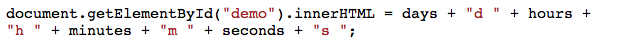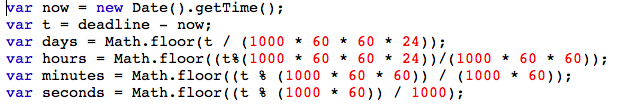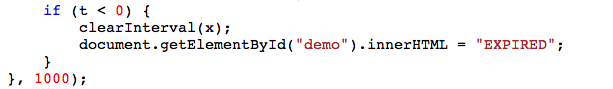# countdown in Javascript

Posted by  Franco Falkov
509  View(s)
Ratings:
Rate this:

How To Create A Countdown Timer Using JavaScript ?

1.##### Re: countdown in Javascript

How To Create A Countdown Timer Using JavaScript :Basics of a countdown timer are :

-Set a valid end date

-Calculate the time remaining

-Convert the time to a usable format

-Output the clock data as a reusable object

-Display the clock on the page, and stop the clock when it reaches zero

Set a Valid End Date :

`var deadline = new Date("12 july, 2018, 15:55:28").getTime(); `

Calculate Remaining Time :Output the result:Write some text if the countdown is over:```<!DOCTYPE HTML>
<html>
<style>
p {
text-align: center;
font-size: 60px;
}
</style>
<body>
<p id="demo"></p>
<script>
var deadline = new Date("July 12, 2018 15:37:25").getTime();
var x = setInterval(function() {
var now = new Date().getTime();
var t = deadline - now;
var days = Math.floor(t / (1000 * 60 * 60 * 24));
var hours = Math.floor((t%(1000 * 60 * 60 * 24))/(1000 * 60 * 60));
var minutes = Math.floor((t % (1000 * 60 * 60)) / (1000 * 60));
var seconds = Math.floor((t % (1000 * 60)) / 1000);
document.getElementById("demo").innerHTML = days + "d "
+ hours + "h " + minutes + "m " + seconds + "s ";
if (t < 0) {
clearInterval(x);
document.getElementById("demo").innerHTML = "EXPIRED";
}
}, 1000);
</script>
```